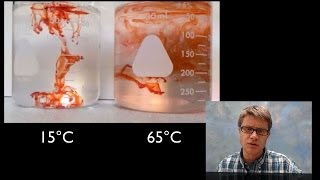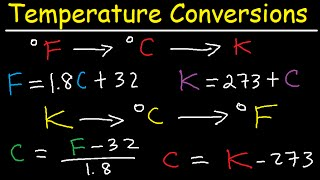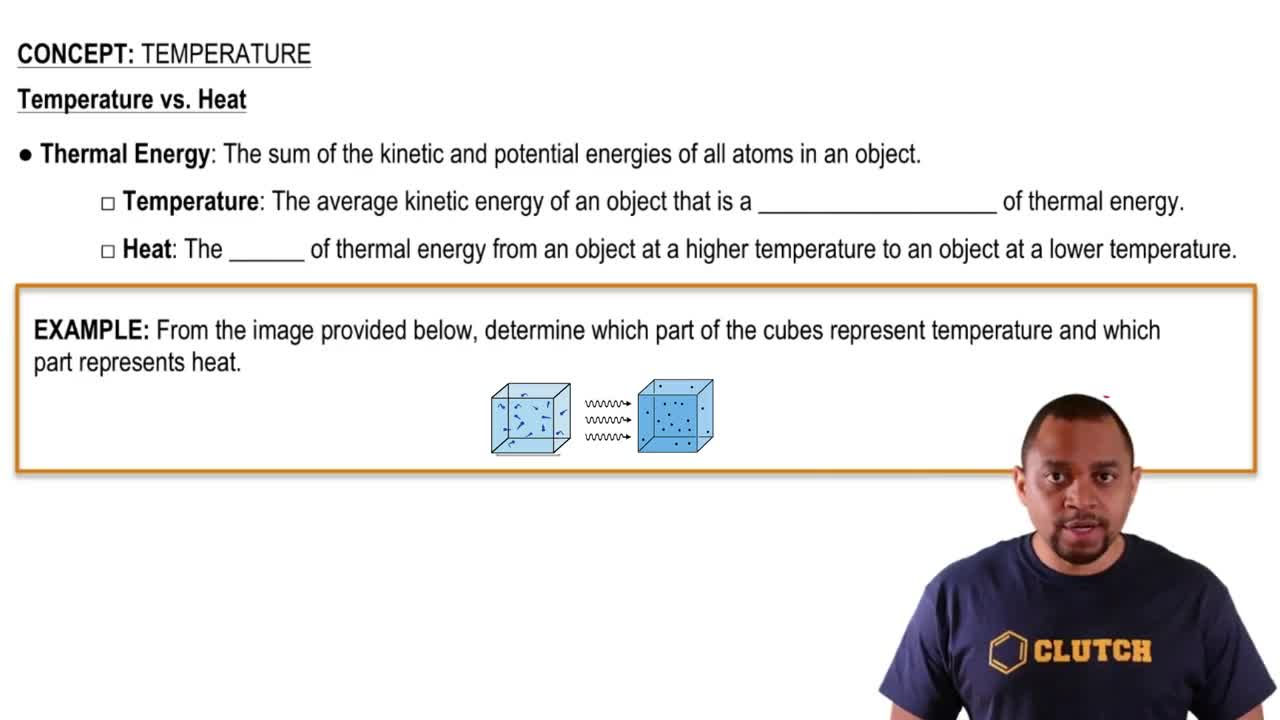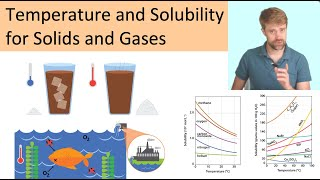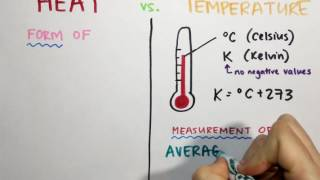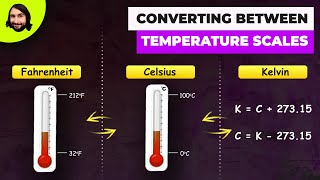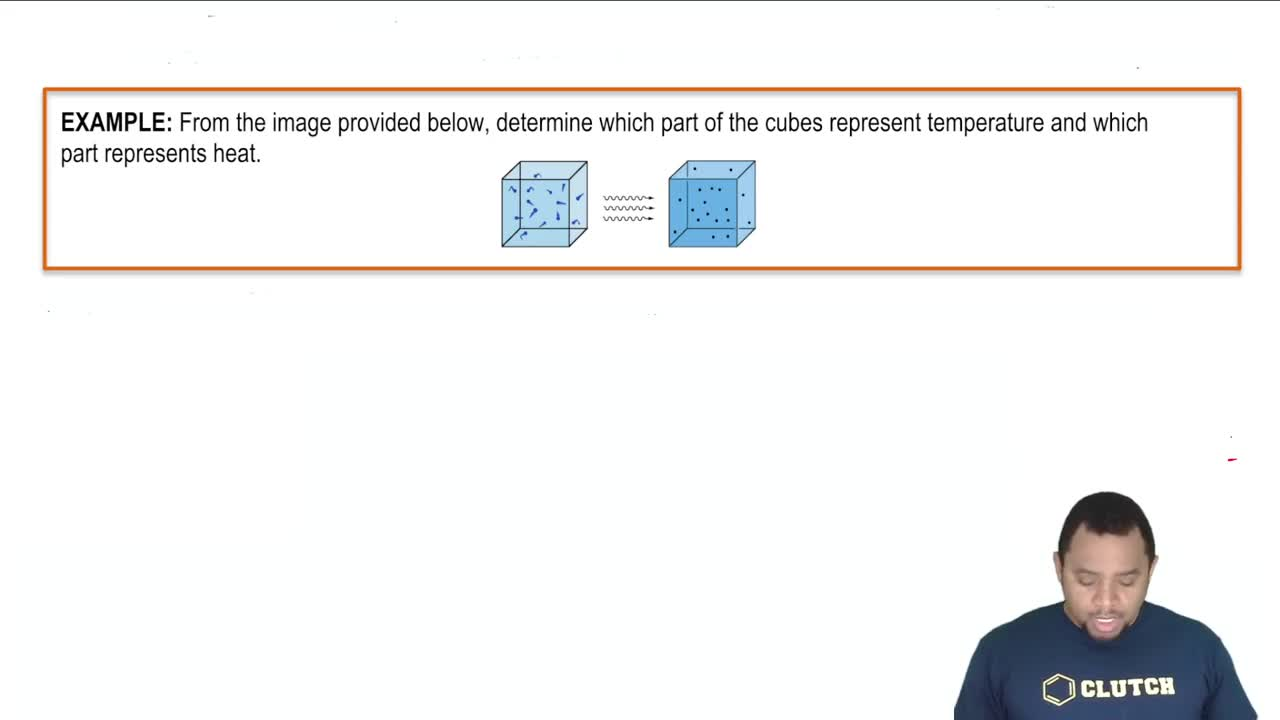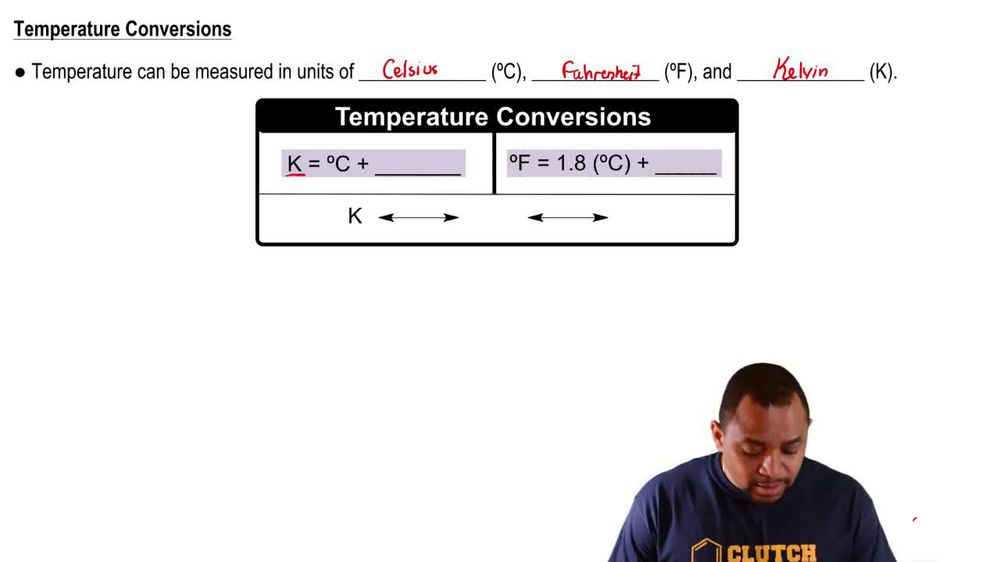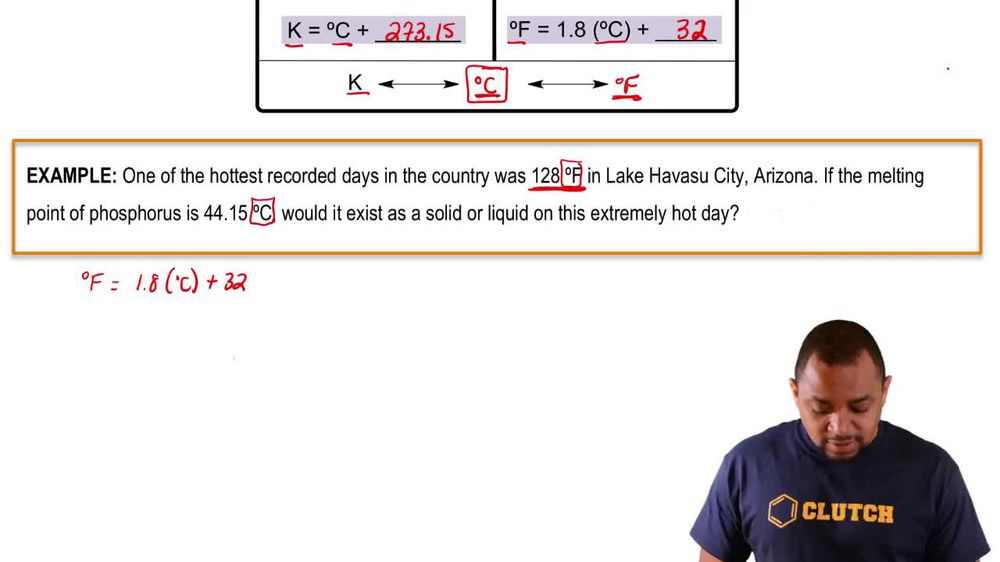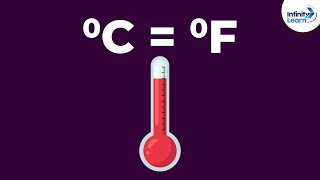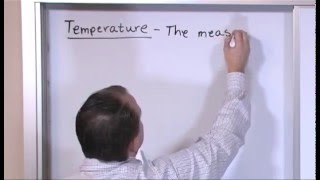Start typing, then use the up and down arrows to select an option from the list.
1. 1. Intro to General Chemistry2. Temperature
Problem

# Tungsten, the element used to make filaments in light bulbs, has a melting point of 6192 °F. Convert this temperature to degrees Celsius and to kelvin.

Relevant Solution1m
Play a video:
Hey everyone. So we're going to practice converting our temperatures specifically from degrees Fahrenheit into degrees Celsius and then finally into Calvin. So we're given an average temperature of 13°F. So first let's think about the formula that we know. So we know that in order to convert this, we have degrees Celsius is equal two F -32 in parentheses and we're going to multiply that by 5/9. So plugging in our values, we get 13°F 32 Times 5/9. And this will get us to a value of -10. and this is going to be in degrees Celsius. Now to convert into kelvin, we know that we have to add 273. and that will get us to a final value of 262.59 Kelvin, Which we can definitely round up to 263 Kelvin, which is going to be answer choice B So I hope that made sense. And let us know if you have any questions